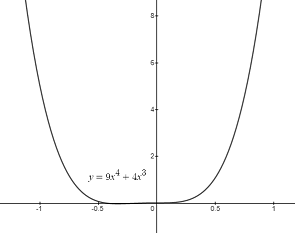# Find the critical points and determine if the function is increasing or depressing on the given...

## Question:

Find the critical points and determine if the function is increasing or depressing on the given intervals {eq}\displaystyle y = 9x^4 + 4x^3 {/eq}

## Critical Point of Function:

We have to find the critical points and determine in which intervals the function is increasing or decreasing? Critical point can be find by equation the first derivative of the function to zero, we find the critical points. With the help of the graph we can see the desired result.

## Answer and Explanation:

The given function is{eq}y = 9x^4 + 4x^3. {/eq}

Find the first derivative of the function and equate them to zero so, we have \begin{align*} y &= 9{x^4} + 4{x^3}\\ \frac{{dy}}{{dx}} &= \frac{d}{{dx}}\left[ {9{x^4} + 4{x^3}} \right]\\ \frac{{dy}}{{dx}} &= 36{x^3} + 12{x^2}\\ \frac{{dy}}{{dx}} &= 12{x^2}\left( {3x + 1} \right)\\ 0 &= 12{x^2}\left( {3x + 1} \right)\\ x &= 0,x = - \frac{1}{3}. \end{align*}

So, the critical points are at {eq}x= 0, - \frac{1}{3} {/eq}. The graph:From the graph, we can say that when {eq}- \infty < x < - \frac{1}{3} {/eq}, the first derivative is negative, the function is decreasing. When {eq}- \frac{1}{3} <x<0 {/eq}, the first derivative is positive, the function is increasing.When {eq}0 < x < \infty {/eq}, the first derivative is positive, the function is increasing.

Therefore, in interval {eq}- \infty < x < - \frac{1}{3} {/eq} it is deceasing. At {eq}- \frac{1}{3} <x<\infty {/eq} it is increasing.

#### Learn more about this topic:Finding Critical Points in Calculus: Function & Graph

from CAHSEE Math Exam: Tutoring Solution

Chapter 8 / Lesson 9
163K